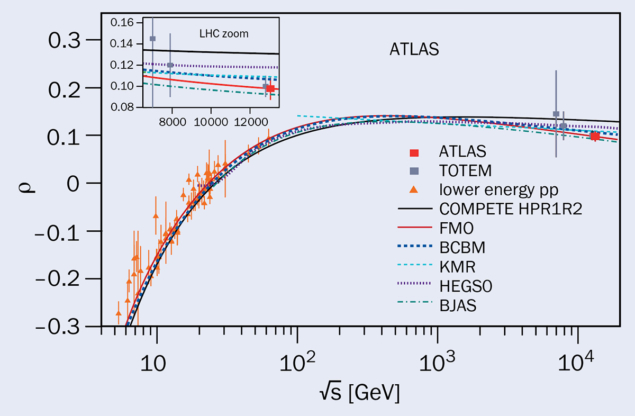# Probing QCD beyond LHC energies

7 November 2022

A report from the ATLAS experiment.Fig. 1. The measured differential elastic proton–proton cross section as a function of the Mandelstam t variable together with a fit function used to extract the physics parameters. Source: arXiv:2207.12246

The study of elastic hadron scattering is a cornerstone in understanding the non-perturbative properties of strong interactions. A key role is played by experiments at the LHC, where it is possible to precisely measure proton–proton (pp) interactions at a very high centre-of-mass energy. The goal is to detect the process pp  pp, in which the interacting protons remain intact and are scattered at angles of a few microradians with respect to the beamline. The importance of such measurements follows from their relation to the total hadronic pp cross section via the optical theorem, and to properties of proton interactions at asymptotically high energies via dispersion relations.

In ATLAS, elastic scattering is studied using a dedicated experimental setup – the ALFA detectors, which allow measurements of scattered-proton trajectories inside the beam pipe, just a few millimetres from the LHC beam. They are installed inside so-called Roman pots located at distances of 237 and 245 m on either side of the ATLAS interaction point.

Recently, ATLAS reported a measurement of elastic scattering at a centre-of-mass energy of 13 TeV. The data were collected with a special setting of the LHC magnets characterised by a high β* of 2500 m, which results in a large beam-spot size and a very small beam divergence. The latter allows precise measurements of small scattering angles. With these optics, the ALFA system detected events characterised by very small values of the Mandelstam t variable, which is proportional to the scattering angle squared. Measurements of small |t| values give access to the Coulomb–nuclear interference (CNI) kinematic region, where the contribution from electromagnetic and strong interactions are of similar magnitude.

The ALFA detectors use scintillating- fibre technology to measure the position of the passing proton. The t value for each event is reconstructed from the measured positions using knowledge of the magnetic fields of the LHC magnets between the interaction point and the detectors. The selection of candidate events is based on the strong correlations between the elastically scattered protons, resulting from energy and momentum conservation. The analysis is heavily based on data-driven techniques, which are used for the alignment of the detectors, background estimation, evaluation of reconstruction efficiency and optics tuning.

Figure 1 presents the measured differential elastic cross section as a function of t. The shape of the distribution is sensitive to important physics parameters, such as the total cross section (σtot) and the ρ parameter, defined as the ratio of real to imaginary parts of the forward scattering amplitude. The smallest |t| values, and thus the smallest scattering angles, are dominated by the electromagnetic interaction between the protons. The CNI effects are strongest for |t| around 10–3 GeV2 and provide the sensitivity to the ρ parameter. For larger |t| values, the strong interaction dominates, and the spectrum depends on the value of σtot. The physics parameters are extracted from a fit to the t distribution.Fig. 2. The centre-of-mass energy evolution of the ρ parameter together with predictions of various theoretical models. Source: arXiv:2207.12246

The ρ parameter is related, through dispersion relations, to the energy dependence of σtot, with a certain sensitivity also to energies above those at the LHC. In addition, ρ is sensitive to possible differences between pp and pp scattering amplitudes at asymptotic energies. ATLAS measured ρ = 0.098 ± 0.011, in agreement with a previous TOTEM measurement. The result is in conflict with pre-LHC theoretical expectations (see the COMPETE line in figure 2), which assumed that no pp/pp  difference is present asymptotically and that σtot increases proportionally to the squared logarithm of the centre-of-mass energy, similarly to the evolution observed at accessible energies back then. This suggests that one of the above assumptions is incorrect: either the increase of σtot slows down above LHC energies, or protons and antiprotons interact differently at asymptotic energies. The second statement is often associated with the so-called odderon exchange. Both possibilities affect our understanding of the high-energy behaviour of strong interactions.

ATLAS also measured the total pp hadronic cross section σtot = (104.7 ± 1.1) mb. This is the most precise measurement to date at this energy, due to a dedicated luminosity measurement that contributed less than 1 mb to the total systematic uncertainty. However, the long-standing tension between the ATLAS and TOTEM σtot measurements, with the latter being about 5% higher than ATLAS, persists.

ATLAS has collected more elastic scattering data in LHC Run 2, which are currently being analysed. New data taking is planned during Run 3, where a special run is foreseen at a centre-of-mass energy of 13.6 TeV.